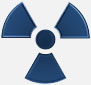## Random Number Generator Usage

As each radiation particle is detected by Geiger Counter, a new random number is generated and displayed on LCD. At the same time number is sent out via TTL serial output. than be accessed by the 3.5 mm jack on the back of DMAD Module. This allows any system to take the random numbers generated by this module for further use. PC can be connected to this module with optional USB-TTL cable and a PC program to read random numbers generated. Serial Data format is 9600 Baud, 8N1 with a byte wide data for random number.

Random Number Generation Theory

True random numbers are useful for data encryption (cryptography), statistical mechanics, probability, gaming, neural networks and disorder systems, to name a few.

Random numbers generated by a computer are notoriously random in an exact defined pattern. So much so that they are many times referred to as pseudo-random numbers. The random numbers generated by this module are truly random.

The way the RNG module operates is simple to understand. However, its fundamental function is best described using a mechanical analogy. Imagine a sequence of numbers painted on the outer edge of a revolving wheel. There is a pointer on the outside of the wheel that points to the number at the top of the wheel. The wheel is set into motion, spinning very rapidly. Then at any given “random” moment in time the wheel is instantly stopped, and the number under the pointer becomes our random number. Once read, the wheel is set back into motion again for next random number generation.

The electronics follow pretty close to the mechanical analogy. The microcontroller spins the sequence of numbers internally. The random point in time when the sequence of numbers is instantly stopped is generated by the detection of a radioactive particle by the Geiger Counter. Background radiation is an ideal quantum mechanical random time-delay generator because it is impossible to predict with any accuracy the exact moment a radioactive particle will be detected. When a particle is detected, a positive pulse is sent to the Digital out of Analog Geiger Counter / Audio out of Digital Geiger Counter. This module is connected to Digital out of Analog Geiger Counter / Audio out of Digital Geiger Counter. On RNG Module, the positive pulse is connected to microcontroller input pin. This positive pulse generates an interrupt in the microcontroller that stops the numbers from spinning. The number is read, displayed and sent out serially on the TTL port.

We determine how many numbers are painted on the wheel by setting the jumpers on the back of this module i.e. setting the range of random number being generated.

There is a simple test to find the trueness of random numbers. If you add all the random numbers that are generated (let’s call this number N) and divide that by number of samples you added together (Let’s call this number S) the answer should be approximately 50% of your number range (Lets call this number R).

For example using a range of 1 – 10, so R = 10. The number of random number samples, is 100, so S = 100. We add those 100 random numbers together, that equals N. So N/S = .5 R In this case the number N when divided by S (100) should equal approximately 5. This would show an even distribution of random numbers though out the range of 1 - 10.

Following link has a test program that will read a file of random numbers and analyze the randomness of the numbers in the file. http://www.fourmilab.ch/random/ We have not tested random numbers generated by this module with the above test program.

Background radiation consists of three sources; Cosmic radiation from the sun and stars. Terrestrial radiation from low levels of uranium, thorium, and their decay products in the soil, air and water. Internal radiation from radioactive potassium-40, carbon-14, lead-210, and other iso-topes found inside our bodies.